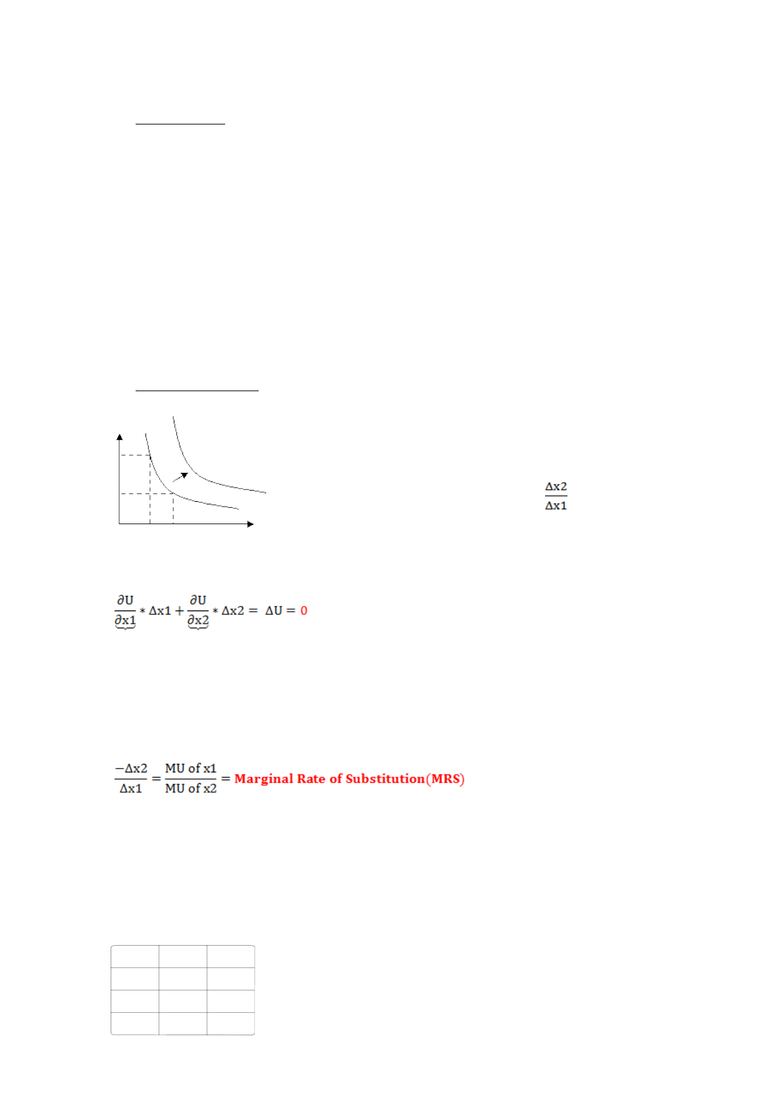# ECO206Y1 Lecture Notes - Indifference Curve, Utility, Substitute Good

47 views3 pages
School
Department
Course
ProfessorUtility Function: CU = u(x1, x2, x3, … , xn)
Math representation of preference ordering:
a.
X>Y U(x)>U(y)
b.
XY U(x)=U(y)
* numbers in the utility function are “ordinal” not “cardinal”*
* These utility functions are only unique to a “positive monotonic transformation”.
e.g. V[U(x) = [U(X)]2; 3 + 2U(X); 5 + lnU(X)
-- Positive monotonic transformation” is like “relabeling of the preferences”;
-- It does not change the order of preferences;
Indifference Set/curve
Set of commodity bundles that satisfies U(x1, x2) = Uo(some level of utility)
x1
x2
-- Indifference curve pass to the right indicate higher utility
(assume “more is better”)
-- Slope of the indifference curve:
1.
Total Differentiate U(x1, x2) = Uo
MU of x1 MU of x2
*MU of x1: the utility one gets from consuming one more unit of x1, holding x2 constant.
Implication:
Measure the rate at which the consumer would be willing to substitute one good for another
e.g.
Suppose U(x1, x2) = x10.5 * x20.5
MRS (x2for x1) = U1/U2 = x2/x1
Assume U=100.
X1
X2
MRS
100
100
1
225
44.44
0.1975
400
25
0.0625
Topic 2 - Utility and Indifference Curves
September 21, 2011
10:29 AM
Unlock document

This preview shows page 1 of the document.
Unlock all 3 pages and 3 million more documents.

## Document Summary

Utility function: cu = u(x1, x2, x3, , xn) Math representation of preference ordering: x>y u(x)>u(y, x y u(x)=u(y) * numbers in the utility function are ordinal not cardinal * From the utility function, we only know the order of the preference (whether x is before y), not the strength of the preference (whether the utility of x is 3 times greater than y) * these utility functions are only unique to a positive monotonic transformation . e. g. v[u(x) = [u(x)]2; 3 + 2u(x); 5 + lnu(x) - positive monotonic transformation is like relabeling of the preferences ; - it does not change the order of preferences; Set of commodity bundles that satisfies u(x 1, x2) = uo (some level of utility) x2. - indifference curve pass to the right indicate higher utility (assume more is better ) *mu of x1: the utility one gets from consuming one more unit of x1, holding x2 constant.

# Get access

\$10 USD/m
Billed \$120 USD annually
Homework Help
Class Notes
Textbook Notes